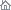Home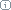About Us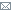ContactBlogWhat's NewProductsBuy NowDownloadsForumGeneXproTools Online Guide Learn how to use the 5 modeling platforms of GeneXproTools with the Online GuideLast update: February 19, 2014Built-in Boolean Functions

Below are listed all the 258 built-in logical functions available in GeneXproTools, including their class (universal logical modules are identified by ULM, whereas universal NAND-like modules are identified by NLM), their representation in Karva Notation (shown in red) and their logical definition (for more details about their implementations, see the built-in grammars of GeneXproTools):

Basic logical functions with 1 and 2 inputs:

• Not (Not): A'
• And (ULM) (And): A·B
• Or (ULM) (Or): A+B
• Nand (NLM) (Nand): (A·B)'
• Nor (NLM) (Nor): (A+B)'
• Exclusive or (Xor): A^B
• If and only if (Nxor): (A^B)'

Basic logical functions with 3 inputs:

• And(a,b,c) (ULM) (And3): A·B·C
• Or(a,b,c) (ULM) (Or3): A+B+C
• Nand with 3 inputs (NLM) (Nand3): (A·B·C)'
• Nor with 3 inputs (NLM) (Nor3): (A+B+C)'
• Odd-3-parity (Odd3): Odd-3-parity
• Even-3-parity (Even3): Even-3-parity

Basic logical functions with 4 inputs:

• And(a,b,c,d) (ULM) (And4): A·B·C·D
• Or(a,b,c,d) (ULM) (Or4): A+B+C+D
• Nand with 4 inputs (NLM) (Nand4): (A·B·C·D)'
• Nor with 4 inputs (NLM) (Nor4): (A+B+C+D)'
• Odd-4-parity (Odd4): Odd-4-parity
• Even-4-parity (Even4): Even-4-parity

Additional logical functions with 1 input:

• Identity function (Id): Id(A) = A
• Constant zero function (Zero): 0(A) = 0
• Constant one function (One): 1(A) = 1

Additional logical functions with 2 inputs:

• Less than (ULM) (LT): A < B
• Greater than (ULM) (GT): A > B
• Less or equal (ULM) (LOE): A <= B
• Greater or equal (ULM) (GOE): A >= B
• Not A (NotA): NOT(A,B) = NOT A
• Not B (NotB): NOT(A,B) = NOT B
• Input A (IdA): IdA(A,B) = A
• Input B (IdB): IdB(A,B) = B
• 0(a,b) (Zero2): 0(A,B) = 0
• 1(a,b) (One2): 1(A,B) = 1

Derived logical functions with 3 inputs:

• LT(a,b,c) (ULM) (LT3): A < B < C
• GT(a,b,c) (ULM) (GT3): A > B > C
• LOE(a,b,c) (ULM) (LOE3): A <= B <= C
• GOE(a,b,c) (ULM) (GOE3): A >= B >= C

Common logical functions with 3 inputs:

• 3-Multiplexer (ULM) (Mux): 3-Multiplexer
• If(a,b,c) (ULM) (If): If A = 1, then B, else C
• Majority(a,b,c) (ULM) (Maj): Majority(A,B,C)
• Minority(a,b,c) (ULM) (Min): Minority(A,B,C)
• Exactly two off (ULM) (2Off): Exactly two off
• Exactly two on (ULM) (2On): Exactly two on

Universal logical modules with 3 inputs (series A):

• ULM with 3 inputs (A1) (LM3A1): AC'+BC
• ULM with 3 inputs (A2) (LM3A2): AC'+B'C
• ULM with 3 inputs (A3) (LM3A3): A'C'+BC
• NLM with 3 inputs (A4) (LM3A4): A'C'+B'C

Universal logical modules with 3 inputs (series B):

• ULM with 3 inputs (B1) (LM3B1): (A+C')·(B+C)
• ULM with 3 inputs (B2) (LM3B2): (A+C')·(B'+C)
• ULM with 3 inputs (B3) (LM3B3): (A'+C')·(B+C)
• NLM with 3 inputs (B4) (LM3B4): (A'+C')·(B'+C)

Universal logical modules with 3 inputs (series C):

• ULM with 3 inputs (C1) (LM3C1): AB'+BC
• ULM with 3 inputs (C2) (LM3C2): AB'+ BC'
• ULM with 3 inputs (C3) (LM3C3): A'B'+BC
• NLM with 3 inputs (C4) (LM3C4): A'B'+ BC'

Universal logical modules with 3 inputs (series D):

• ULM with 3 inputs (D1) (LM3D1): (A+B')·(B+C)
• ULM with 3 inputs (D2) (LM3D2): (A+B')·(B+C')
• ULM with 3 inputs (D3) (LM3D3): (A'+B')·(B+C)
• NLM with 3 inputs (D4) (LM3D4): (A'+B')·(B+C')

Universal logical modules with 3 inputs (series E):

• ULM with 3 inputs (E1) (LM3E1): A'C+ AB'
• ULM with 3 inputs (E2) (LM3E2): A'C'+AB
• NLM with 3 inputs (E3) (LM3E3): A'C'+ AB'

Universal logical modules with 3 inputs (series F):

• ULM with 3 inputs (F1) (LM3F1): (A'+C)·(A+B')
• ULM with 3 inputs (F2) (LM3F2): (A'+C')·(A+B)
• NLM with 3 inputs (F3) (LM3F3): (A'+C')·(A+B')

Universal logical modules with 3 inputs (series G):

• ULM with 3 inputs (G1) (LM3G1): (A^C')·(B^C)
• ULM with 3 inputs (G2) (LM3G2): (A^C')·(B'^C)
• ULM with 3 inputs (G3) (LM3G3): (A'^C')·(B^C)
• ULM with 3 inputs (G4) (LM3G4): (A'^C')·(B'^C)

Universal logical modules with 3 inputs (series H):

• ULM with 3 inputs (H1) (LM3H1): ((A·B)'· C)'
• ULM with 3 inputs (H2) (LM3H2): (A·(B·C)')'
• ULM with 3 inputs (H3) (LM3H3): ((A+B)'+ C)'
• ULM with 3 inputs (H4) (LM3H4): (A+(B+C)')'

Comparison IF THEN ELSE functions with 3 inputs (series A):

• Less Than with 3 inputs (A) (NLM) (LT3A): If A < B, then (A·C), else (B·C)'
• Greater Than with 3 inputs (A) (NLM) (GT3A): If A > B, then (A·C), else (B·C)'
• Less Or Equal To with 3 inputs (A) (ULM) (LOE3A): If A <= B, then (A·C), else (B·C)'
• Greater Or Equal To with 3 inputs (A) (ULM) (GOE3A): If A >= B, then (A·C), else (B·C)'
• Equal To with 3 inputs (A) (ULM) (ET3A): If A = B, then (A·C), else (B·C)'
• Not Equal To with 3 inputs (A) (NLM) (NET3A): If A != B, then (A·C), else (B·C)'

Comparison IF THEN ELSE functions with 3 inputs (series B):

• Less Than with 3 inputs (B) (ULM) (LT3B): If A < B, then (A·C)', else (A·C)
• Greater Than with 3 inputs (B) (ULM) (GT3B): If A > B, then (A·C)', else (A·C)
• Less Or Equal To with 3 inputs (B) (NLM) (LOE3B): If A <= B, then (A·C)', else (A·C)
• Greater Or Equal To with 3 inputs (B) (NLM) (GOE3B): If A >= B, then (A·C)', else (A·C)
• Equal To with 3 inputs (B) (NLM) (ET3B): If A = B, then (A·C)', else (A·C)
• Not Equal To with 3 inputs (B) (ULM) (NET3B): If A != B, then (A·C)', else (A·C)

Comparison IF THEN ELSE functions with 3 inputs (series C):

• Less Than with 3 inputs (C) (ULM) (LT3C): If A < B, then (A+C)', else (B+C)
• Greater Than with 3 inputs (C) (ULM) (GT3C): If A > B, then (A+C)', else (B+C)
• Less Or Equal To with 3 inputs (C) (NLM) (LOE3C): If A <= B, then (A+C)', else (B+C)
• Greater Or Equal To with 3 inputs (C) (NLM) (GOE3C): If A >= B, then (A+C)', else (B+C)
• Equal To with 3 inputs (C) (NLM) (ET3C): If A = B, then (A+C)', else (B+C)
• Not Equal To with 3 inputs (C) (ULM) (NET3C): If A != B, then (A+C)', else (B+C)

Additional universal logical modules with 3 inputs:

• Rule 4 (ULM) (T004): 00000100
• Rule 8 (ULM) (T008): 00001000
• Rule 9 (NLM) (T009): 00001001
• Rule 32 (ULM) (T032): 00100000
• Rule 33 (NLM) (T033): 00100001
• Rule 41 (NLM) (T041): 00101001
• Rule 55 (NLM) (T055): 00110111
• Rule 57 (NLM) (T057): 00111001
• Rule 64 (ULM) (T064): 01000000
• Rule 65 (NLM) (T065): 01000001
• Rule 69 (NLM) (T069): 01000101
• Rule 73 (NLM) (T073): 01001001
• Rule 81 (NLM) (T081): 01010001
• Rule 89 (NLM) (T089): 01011001
• Rule 93 (NLM) (T093): 01011101
• Rule 96 (ULM) (T096): 01100000
• Rule 101 (NLM) (T101): 01100101
• Rule 109 (NLM) (T109): 01101101
• Rule 111 (NLM) (T111): 01101111
• Rule 121 (NLM) (T121): 01111001
• Rule 123 (NLM) (T123): 01111011
• Rule 125 (NLM) (T125): 01111101
• Rule 154 (ULM) (T154): 10011010
• Rule 223 (ULM) (T223): 11011111
• Rule 239 (ULM) (T239): 11101111
• Rule 249 (ULM) (T249): 11111001
• Rule 251 (ULM) (T251): 11111011
• Rule 253 (ULM) (T253): 11111101

Derived logical functions with 4 inputs:

• LT(a,b,c,d) (ULM) (LT4): A < B < C < D
• GT(a,b,c,d) (ULM) (GT4): A > B > C > D
• LOE(a,b,c,d) (ULM) (LOE4): A <= B <= C <= D
• GOE(a,b,c,d) (ULM) (GOE4): A >= B >= C >= D

Common logical functions with 4 inputs:

• Tie (ULM) (Tie): Tie
• Not tie (ULM) (Ntie): Not tie
• Exactly three off (ULM) (3Off): Exactly three off
• Exactly three on (ULM) (3On): Exactly three on

Universal logical modules with 4 inputs (series A):

• ULM with 4 inputs (A1) (LM4A1): AD'+BD+CD
• ULM with 4 inputs (A2) (LM4A2): AD'+B'D+CD
• ULM with 4 inputs (A3) (LM4A3): AD'+BD+C'D
• ULM with 4 inputs (A4) (LM4A4): AD'+B'D+C'D
• ULM with 4 inputs (A5) (LM4A5): A'D'+BD+CD
• ULM with 4 inputs (A6) (LM4A6): A'D'+B'D+CD
• ULM with 4 inputs (A7) (LM4A7): A'D'+BD+C'D
• NLM with 4 inputs (A8) (LM4A8): A'D'+B'D+C'D

Universal logical modules with 4 inputs (series B):

• ULM with 4 inputs (B1) (LM4B1): (A+D')·(B+D)·(C+D)
• ULM with 4 inputs (B2) (LM4B2): (A+D')·(B'+D)·(C+D)
• ULM with 4 inputs (B3) (LM4B3): (A+D')·(B+D)·(C'+D)
• ULM with 4 inputs (B4) (LM4B4): (A+D')·(B'+D)·(C'+D)
• ULM with 4 inputs (B5) (LM4B5): (A'+D')·(B+D)·(C+D)
• ULM with 4 inputs (B6) (LM4B6): (A'+D')·(B'+D)·(C+D)
• ULM with 4 inputs (B7) (LM4B7): (A'+D')·(B+D)·(C'+D)
• NLM with 4 inputs (B8) (LM4B8): (A'+D')·(B'+D)·(C'+D)

Universal logical modules with 4 inputs (series C):

• ULM with 4 inputs (C1) (LM4C1): AB'+BC+BD
• ULM with 4 inputs (C2) (LM4C2): AB'+B'C+BD
• ULM with 4 inputs (C3) (LM4C3): AB'+BC+B'D
• ULM with 4 inputs (C4) (LM4C4): AB'+B'C+B'D
• ULM with 4 inputs (C5) (LM4C5): A'B'+BC+BD
• ULM with 4 inputs (C6) (LM4C6): A'B'+B'C+BD
• ULM with 4 inputs (C7) (LM4C7): A'B'+BC+B'D
• NLM with 4 inputs (C8) (LM4C8): A'B'+B'C+B'D

Universal logical modules with 4 inputs (series D):

• ULM with 4 inputs (D1) (LM4D1): (A+B')·(B+C)·(B+D)
• ULM with 4 inputs (D2) (LM4D2): (A+B')·(B'+C)·(B+D)
• ULM with 4 inputs (D3) (LM4D3): (A+B')·(B+C)·(B'+D)
• ULM with 4 inputs (D4) (LM4D4): (A+B')·(B'+C)·(B'+D)
• ULM with 4 inputs (D5) (LM4D5): (A'+B')·(B+C)·(B+D)
• ULM with 4 inputs (D6) (LM4D6): (A'+B')·(B'+C)·(B+D)
• ULM with 4 inputs (D7) (LM4D7): (A'+B')·(B+C)·(B'+D)
• NLM with 4 inputs (D8) (LM4D8): (A'+B')·(B'+C)·(B'+D)

Universal logical modules with 4 inputs (series E):

• ULM with 4 inputs (E1) (LM4E1): AC'+BC+CD
• ULM with 4 inputs (E2) (LM4E2): AC'+B'C+CD
• ULM with 4 inputs (E3) (LM4E3): AC'+BC+C'D
• ULM with 4 inputs (E4) (LM4E4): AC'+B'C+C'D
• ULM with 4 inputs (E5) (LM4E5): A'C'+BC+CD
• ULM with 4 inputs (E6) (LM4E6): A'C'+B'C+CD
• ULM with 4 inputs (E7) (LM4E7): A'C'+BC+C'D
• NLM with 4 inputs (E8) (LM4E8): A'C'+B'C+C'D

Universal logical modules with 4 inputs (series F):

• ULM with 4 inputs (F1) (LM4F1): (A+C')·(B+C)·(C+D)
• ULM with 4 inputs (F2) (LM4F2): (A+C')·(B'+C)·(C+D)
• ULM with 4 inputs (F3) (LM4F3): (A+C')·(B+C)·(C'+D)
• ULM with 4 inputs (F4) (LM4F4): (A+C')·(B'+C)·(C'+D)
• ULM with 4 inputs (F5) (LM4F5): (A'+C')·(B+C)·(C+D)
• ULM with 4 inputs (F6) (LM4F6): (A'+C')·(B'+C)·(C+D)
• ULM with 4 inputs (F7) (LM4F7): (A'+C')·(B+C)·(C'+D)
• NLM with 4 inputs (F8) (LM4F8): (A'+C')·(B'+C)·(C'+D)

Universal logical modules with 4 inputs (series G):

• ULM with 4 inputs (G1) (LM4G1): A'D+AB+AC
• ULM with 4 inputs (G2) (LM4G2): A'D+AB'+AC
• ULM with 4 inputs (G3) (LM4G3): A'D+AB+ AC'
• ULM with 4 inputs (G4) (LM4G4): A'D+AB'+ AC'
• ULM with 4 inputs (G5) (LM4G5): A'D'+AB+AC
• ULM with 4 inputs (G6) (LM4G6): A'D'+AB'+AC
• ULM with 4 inputs (G7) (LM4G7): A'D'+AB+ AC'
• NLM with 4 inputs (G8) (LM4G8): A'D'+AB'+ AC'

Universal logical modules with 4 inputs (series H):

• ULM with 4 inputs (H1) (LM4H1): (A'+D)·(A+B)·(A+C)
• ULM with 4 inputs (H2) (LM4H2): (A'+D)·(A+B')·(A+C)
• ULM with 4 inputs (H3) (LM4H3): (A'+D)·(A+B)·(A+C')
• ULM with 4 inputs (H4) (LM4H4): (A'+D)·(A+B')·(A+C')
• ULM with 4 inputs (H5) (LM4H5): (A'+D')·(A+B)·(A+C)
• ULM with 4 inputs (H6) (LM4H6): (A'+D')·(A+B')·(A+C)
• ULM with 4 inputs (H7) (LM4H7): (A'+D')·(A+B)·(A+C')
• NLM with 4 inputs (H8) (LM4H8): (A'+D')·(A+B')·(A+C')

Universal logical modules with 4 inputs (series I):

• NLM with 4 inputs (I1) (LM4I1): (((A·B)'·C)'· D)'
• NLM with 4 inputs (I2) (LM4I2): (A·(B·(C·D)')')'
• NLM with 4 inputs (I3) (LM4I3): ((A·(B· C)')'·D)'
• NLM with 4 inputs (I4) (LM4I4): (A·((B·C)'· D)')'
• NLM with 4 inputs (I5) (LM4I5): (((A+B)'+C)'+ D)'
• NLM with 4 inputs (I6) (LM4I6): (A+(B+(C+D)')')'
• NLM with 4 inputs (I7) (LM4I7): ((A+(B+ C)')'+D)'
• NLM with 4 inputs (I8) (LM4I8): (A+((B+C)'+ D)')'

Comparison IF THEN ELSE functions with 4 inputs (series A):

• Less Than with 4 inputs (A) (ULM) (LT4A): If A < B, then C, else D
• Greater Than with 4 inputs (A) (ULM) (GT4A): If A > B, then C, else D
• Less Or Equal To with 4 inputs (A) (ULM) (LOE4A): If A <= B, then C, else D
• Greater Or Equal To with 4 inputs (A) (ULM) (GOE4A): If A >= B, then C, else D
• Equal To with 4 inputs (A) (ULM) (ET4A): If A = B, then C, else D
• Not Equal To with 4 inputs (A) (ULM) (NET4A): If A != B, then C, else D

Comparison IF THEN ELSE functions with 4 inputs (series B):

• Less Than with 4 inputs (B) (NLM) (LT4B): If A < B, then (C·D), else D'
• Greater Than with 4 inputs (B) (NLM) (GT4B): If A > B, then (C·D), else D'
• Less Or Equal To with 4 inputs (B) (ULM) (LOE4B): If A <= B, then (C·D), else D'
• Greater Or Equal To with 4 inputs (B) (ULM) (GOE4B): If A >= B, then (C·D), else D'
• Equal To with 4 inputs (B) (ULM) (ET4B): If A = B, then (C·D), else D'
• Not Equal To with 4 inputs (B) (NLM) (NET4B): If A != B, then (C·D), else D'

Comparison IF THEN ELSE functions with 4 inputs (series C):

• Less Than with 4 inputs (C) (NLM) (LT4C): If A < B, then (C+D), else D'
• Greater Than with 4 inputs (C) (NLM) (GT4C): If A > B, then (C+D), else D'
• Less Or Equal To with 4 inputs (C) (ULM) (LOE4C): If A <= B, then (C+D), else D'
• Greater Or Equal To with 4 inputs (C) (ULM) (GOE4C): If A >= B, then (C+D), else D'
• Equal To with 4 inputs (C) (ULM) (ET4C): If A = B, then (C+D), else D'
• Not Equal To with 4 inputs (C) (NLM) (NET4C): If A != B, then (C+D), else D'

Comparison IF THEN ELSE functions with 4 inputs (series D):

• Less Than with 4 inputs (D) (NLM) (LT4D): If A < B, then (A·D), else (C·D)'
• Greater Than with 4 inputs (D) (NLM) (GT4D): If A > B, then (A·D), else (C·D)'
• Less Or Equal To with 4 inputs (D) (ULM) (LOE4D): If A <= B, then (A·D), else (C·D)'
• Greater Or Equal To with 4 inputs (D) (ULM) (GOE4D): If A >= B, then (A·D), else (C·D)'
• Equal To with 4 inputs (D) (ULM) (ET4D): If A = B, then (A·D), else (C·D)'
• Not Equal To with 4 inputs (D) (NLM) (NET4D): If A != B, then (A·D), else (C·D)'

Comparison IF THEN ELSE functions with 4 inputs (series E):

• Less Than with 4 inputs (E) (ULM) (LT4E): If A < B, then (A·D)', else (A·C)
• Greater Than with 4 inputs (E) (ULM) (GT4E): If A > B, then (A·D)', else (A·C)
• Less Or Equal To with 4 inputs (E) (NLM) (LOE4E): If A <= B, then (A·D)', else (A·C)
• Greater Or Equal To with 4 inputs (E) (NLM) (GOE4E): If A >= B, then (A·D)', else (A·C)
• Equal To with 4 inputs (E) (NLM) (ET4E): If A = B, then (A·D)', else (A·C)
• Not Equal To with 4 inputs (E) (ULM) (NET4E): If A != B, then (A·D)', else (A·C)

Additional universal logical modules with 4 inputs:

• Rule 00002 (ULM) (Q0002): 0000000000000010
• Rule 00028 (ULM) (Q001C): 0000000000011100
• Rule 00072 (ULM) (Q0048): 0000000001001000
• Rule 02048 (ULM) (Q0800): 0000100000000000
• Rule 13176 (ULM) (Q3378): 0011001101111000
• Rule 13429 (NLM) (Q3475): 0011010001110101
• Rule 15536 (ULM) (Q3CB0): 0011110010110000
• Rule 15855 (NLM) (Q3DEF): 0011110111101111
• Rule 15871 (NLM) (Q3DFF): 0011110111111111
• Rule 16896 (ULM) (Q4200): 0100001000000000
• Rule 19473 (NLM) (Q4C11): 0100110000010001
• Rule 20736 (ULM) (Q5100): 0101000100000000
• Rule 24303 (NLM) (Q5EEF): 0101111011101111
• Rule 24319 (NLM) (Q5EFF): 0101111011111111
• Rule 27245 (NLM) (Q6A6D): 0110101001101101
• Rule 28533 (NLM) (Q6F75): 0110111101110101
• Rule 29892 (ULM) (Q74C4): 0111010011000100
• Rule 32163 (NLM) (Q7DA3): 0111110110100011
• Rule 33540 (ULM) (Q8304): 1000001100000100
• Rule 33840 (ULM) (Q8430): 1000010000110000
• Rule 34115 (ULM) (Q8543): 1000010101000011
• Rule 40320 (ULM) (Q9D80): 1001110110000000
• Rule 41106 (ULM) (QA092): 1010000010010010
• Rule 45930 (ULM) (QB36A): 1011001101101010
• Rule 52175 (ULM) (QCBCF): 1100101111001111
• Rule 61105 (ULM) (QEEB1): 1110111010110001
• Rule 61439 (ULM) (QEFFF): 1110111111111111
• Rule 65403 (ULM) (QFF7B): 1111111101111011
• Rule 65526 (ULM) (QFFF6): 1111111111110110
• Rule 65531 (ULM) (QFFFB): 1111111111111011

Related Tutorials:

Related Videos:

 Leave Feedback Please enter the number below using the combo boxes before sending your feedback. 3 8 4 0 1 2 3 4 5 6 7 8 9   0 1 2 3 4 5 6 7 8 9   0 1 2 3 4 5 6 7 8 9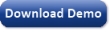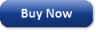Time Limited Trial

Released February 19, 2014

Last update: 5.0.3883New Entries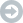Subscribe to the GEP-List

 3 8 4 0 1 2 3 4 5 6 7 8 9   0 1 2 3 4 5 6 7 8 9   0 1 2 3 4 5 6 7 8 9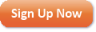Home | What's New | Products | Buy Now | Downloads | Quick Tour | Support | Contact Us | About Gepsoft | Sign UpForum | Blog | Videos | Tutorials | Server Knowledge Base | Logistic Regression Guide | Terms of Use | Privacy & Cookies